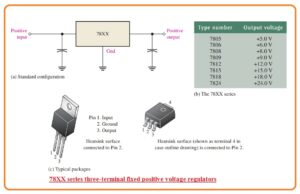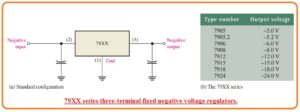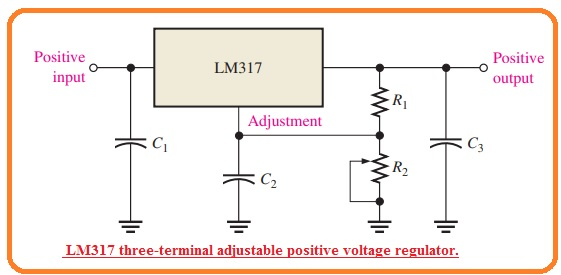Hello, readers welcome to another interesting post. in this post, we will have a detailed look Integrated Circuit Voltage, Regulators. In previous, we discussed the voltage regulator arrangements with the detail. There are numerous linear and switching regulators are exist in the shape of ICs. Normally the linear regulator has 3 terminal which offers positive or negative outputs that have 2 forms fixed or variable.

In this post, we will cover the integrated circuit voltage regulators circuits, operation and some other related factors. So let’s get started with Integrated Circuit Voltage Regulators.

#### What is Fixed Positive Linear Voltage Regulator

• Though there are numerous categories of integrated circuits regulators exist in the market but the series of 78XX of IC regulators is 3 terminals modules that offer the positive value of output.
• The 3 terminal is called input-output and the ground can be seen in below figure.• The last 2 numbers in the part numeric denote the output voltage. For instance, 7805 is type of regulator. For any regulator, the output voltage will be nominal output.
• So the voltage provided by the 7805 can be of range from 4.8 volts to 5.2 volts but has a constant value.
• Other types of output voltage values can be seen in figure denoted as b and their packages is shown in figure denoted as c.
• Capacitors are not common parts but in some cases use at input and output.
• The output capacitors generally behave like a line filter to do improvement in the transit response.
• The input capacitor filters the input and provides prevention from undesired vibrations when the regulators is at some distance apart from the power supply filter so that the line has sufficient value of inductance.
• The 78XX series can generate output current to one ampere when used with sufficient heat sink.
• The input voltage should be almost 2.5 volts over the output voltage for the maintenance of regulation.
• The circuitry has inner thermal overload protection and short circuitry current limiting specification.
• Thermal overload exists when the inner power dissipation is very large and the temperature of the module large than the specific value.
• Every application of regulators needs the device to secured the heat sink to stop thermal overload.

#### Fixed Negative Linear Voltage Regulators

• The 79xx series is a common type of 3 terminal Integrated regulators which offers a fixed negative output value.
• It is the negative voltage counterpart of 78xx series and provides similar features and specifications with the different of pin numbers.
• The below figure denotes the standard arrangement and part numbers and resultant output voltage which exists.#### Adjustable Positive Linear Voltage Regulators

• The LM317 is a common example of 3 terminal positive regulators having variable output capacity.
• The basic arrangement is can be seen in the below figure.• The capacitor are used for decoupling and has not any effect on the dc operation.
• Note that there is an input-output and variable terminal.
• The exterior fixed-resistance and outer variable resistance offer the output voltage variance or adjustment.
• The value of VOut can be changed from 1.2 volts to thirty-seven volts according to the value of resistance.
• The LM317 can offer large then the 1.5 amperes output current to the output.
• LM317 is functioning like a floating regulator since the adjustment terminal is not linked with the ground but floats the value of voltage about the resistance R2.
• It permits the output voltage to be larger than the fixed voltage regulator.

Working of LM317

• In the below figure the constant voltage 1.25 volts reference voltage is retained with the regulator among output point and variable point.
• This constant reference voltage generates a constant current in the resistance R1 irrespective of the value of resistance R2.IREF =VREF/R1=1.25 V/R1

VOUT = VR1 + VR2 = IREFR1 + IREFR2 + IADJR2

= IREF(R1 + R2) + IADJR